Wlodzimierz Bryc Professor of Mathematical SciencesDepartment of Mathematical Sciences University of Cincinnati PO Box 210025 Cincinnati, OH 45221-0025 Fax: (513) 556 3417 Preprints at ArXivBooks Papers Suggested Readings

#### RESEARCH INTERESTS

Probability Theory: non-commutative probability, large deviations, weak dependence, characterizations of stochastic processes.

#### Books and Monographs

1. Normal distribution: characterizations with applications, Lecture Notes in Statistics vol 100, Springer 1995. [DVI 1995] Corrected Version [PDF 2005]

#### PhD Students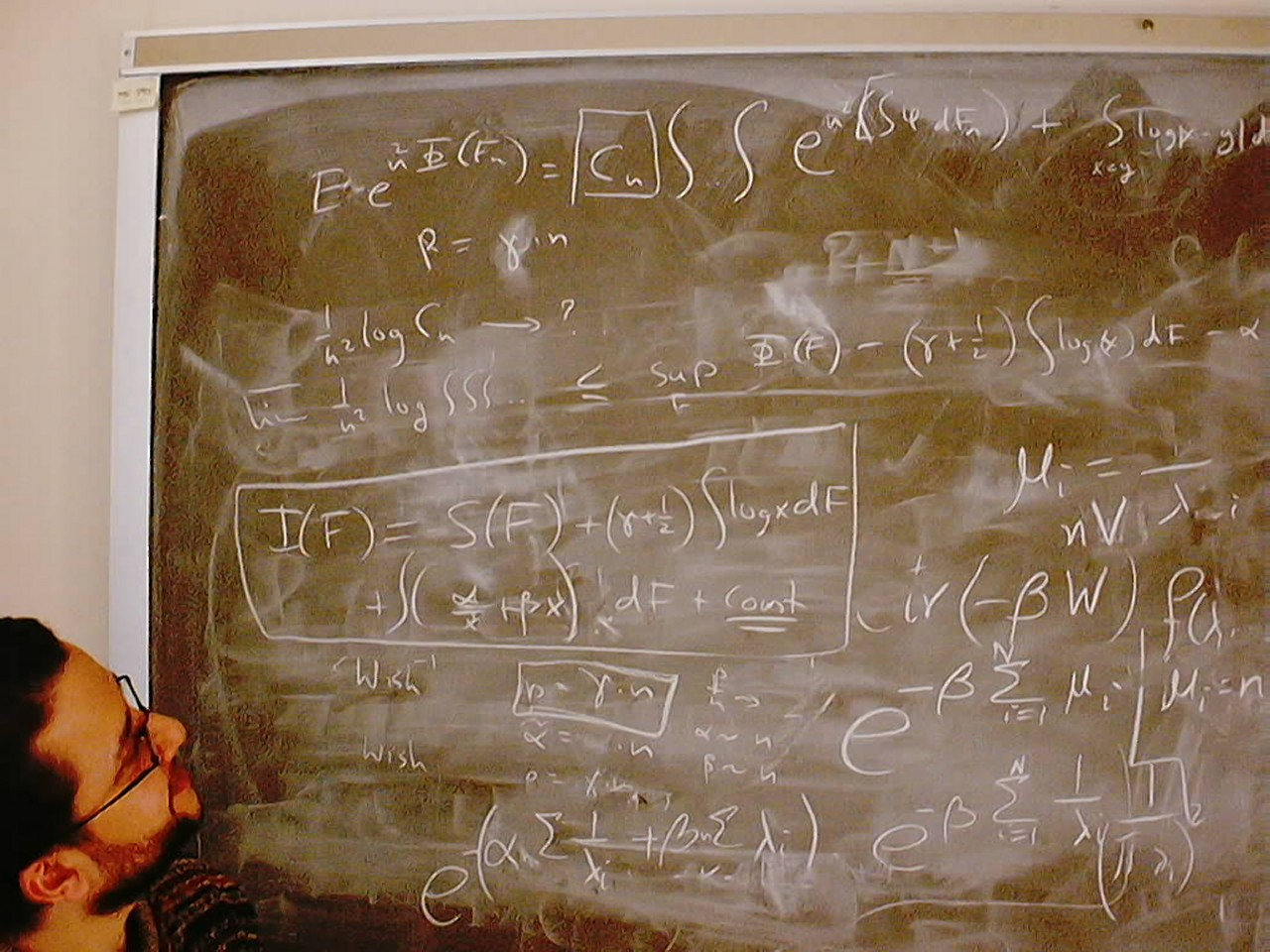Tamer Oraby [PhD 2008] Thesis: Spectra of Random Block-Matrices and Products of Random MatricesAndoniaina Josoa Rarivoarimanana [PhD 2014] Thesis: Unbalanced Urn Models and Applications Your picture here?

#### Math Papers

1.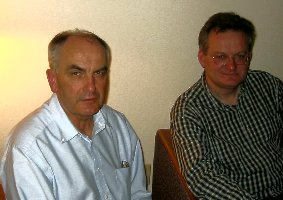On the conditional expectation with respect to a sequence of independent $\sigma$-fields (with S. Kwapien), Z. Wahrscheinlichk. 46 (1979), 221-225.
2. On the approximation theorem of I. Berkes and W. Philipp, Demonstr. Math. 15(1982), 807-816.
3. A remark on continuous independent functions, Ann. Univ. Sci. Budapestinensis 26 (1983), 13-16.
4. On almost sure convergence and integrability of sums of weakly dependent random vectors, Rev. Roumaine de Math. Pures et Appl. 28 (1983), 265-271.
5. Conditional expectation with respect to dependent $\sigma$-fields, Proceedings of VII conference on Probab., Brasov, Romania, 1984, 409-411. (refereed)
6. Multilinear forms and measures of dependence between random variables (with R. Bradley), Journ. Multiv. Anal. 16 (1985), 335-367.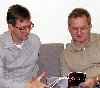7. A characterization of infinite gaussian sequences by conditional moments (with A. Plucinska), Sankhya A vol. 47(1985), 166-173.
8. Some remarks on random vectors with nice enough behaviour of conditional moments, Bull. Polish Acad. Sci. 33(1985), 677-683.
9. Remarks on the foundations of measures of dependence (with R. Bradley & S. Janson), in New Perspectives in Theor. App. Stat, ed. M. Puri, Wiley, 421-437, 1987.
10. On dominations between measures of dependence (with R. Bradley & S. Janson), Journ. Multiv. Anal. 23(1987), 312-329.
11. A characterization of the Poisson process by conditional moments, Stochastics 20(1987), 17-26.
12. Trajectories of Gaussian processes and interpolation of Banach spaces, Stoch. Processes Appl. 25 (1987), 257-263.
13. Remarks on properties of probability distributions determined by conditional moments, Probab. Th. Rel. Fields 78 (1988), 51-62.
14. On the large deviation principle by the asymptotic value method, in: Diffusion Processes and Related Problems in Analysis, Vol I, ed. M. Pinsky, Birkhäuser, Boston 1990, 447-472. (refereed)
15. Some characteristic of normal distribution by conditional moments (with P. Szabowski), Bull Polish Acad. Sci. 38 (1990), 209-218.
16. The Central Limit Theorem for the Tukey's 3R Smoother (with M. Peligrad), Statist. Probab. Lett. 13 (1991), 29-37.17. On the large deviation principle for stationary weakly dependent random fields, Ann. Probab. 20 (1992), 1004-1030.
18. On Large Deviations for Uniformly Strong Mixing Sequences, Stoch. Proc. Appl. 41 (1992), 191-202.
19. On a measure of symmetry for stationary random sequences, Rocky Mount. Math. Journ 22 (1992), 471-476.
20. On bivariate distributions with rotation invariant absolute moments, Sankhya A 54 (1992) 432-439.
21. Moment conditions for almost sure convergence of series of weakly correlated random variables (with W. Smolenski), Proc. Amer. Math. Soc. 119 (1993) 629-635.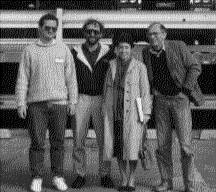22. On the stability problem for conditional expectation (with W. Smolenski), Stat. Probab. Letters. 15 (1992) 41-46.
23. On the large deviation principle for a quadratic functional of the autoregressive process ( with W. Smolenski), Stat. Probab. Letters 17 (1993) 281-285.
24. A remark on the connection between the large deviation principle and the central limit theorem, Stat. Probab. Lett. 18 (1993) 253-256.
25. On probabilities of linear sets (with W. Smolenski), Journ. Mult. Anal. 45(1993) 25-33.
26. On the convergence of averages of mixing sequences (with W. Smolenski), Journ. Theoret. Probab. 6 (1993) 473-483.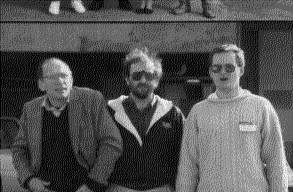27. A sensitivity estimate for Boolean functions, (with W. Smolenski) Computers Math. Applic. 28 (1994) 45-52.
28. On large deviations of empirical measures for stationary Gaussian processes, with A. Dembo, Stoch. Process. Appl. 58 (1995) 23-34.
29. On Gaussian random measure generated by empirical distributions of independent random variables, with W. Smolenski, Demonstratio Mathematica XXVIII (1995) 733-738.
30. Conditional moment representations for dependent random variables Electronic Journal of Probability 1 (1996) paper no 7 pages 1-14 Local Copy
31. Large deviations and strong mixing, with A. Dembo, Annales de l'I.H.P. Probabilitiés & Statistiques 32 (1996) 549-569.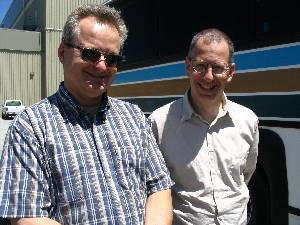32. Large deviations for quadratic functionals of Gaussian process (with A. Dembo) Journal of Theoretical Probability 10 (1997) pp. 307-332 (PDF of Expanded version: IMA Preprint Series No. 1179)
33. Specifying bivariate distributions by polynomial regressions Statistics & Probability Letters, Volume: 47, Issue: 4, May 1, 2000, pp. 391-394
34. Variational representations of Varadhan functionals (with H. Bell) Proc. Amer. Math. Soc. 129 (2001), 2119-2125.35. Stationary fields with linear regressions Ann. Probab. 29 (2001), 504-519
36. Stationary Markov chains with linear regressions, Stoch. Proc. Appl. 93 (2001), pp. 339-348.
37. Classical versions of q-Gaussian processes: conditional moments and Bell's inequality, Comm. Math. Phys., 219 (2001), pp. 259-270.
38.Strong mixing coefficients for non-commutative Gaussian processes (with V. Kaftal). Proc. Amer. Math. Soc. 132 (2004), 523-534.
39. Large deviations of bivariate empirical measures for two systems coupled by a symmetric interaction. Journ. Theoret. Probab. 16 (2003), 935-955.
40. On the maximum correlation coefficient (with A. Dembo and A. Kagan) Theory of Probability and Its Applications Volume 49, (2005) pp. 132-138.
41. Probabilistic aspects of Al-Salam--Chihara polynomials (with W. Matysiak and P. Szablowski) Proc. Amer. Math. Soc. 133 (2005), 1127-1134.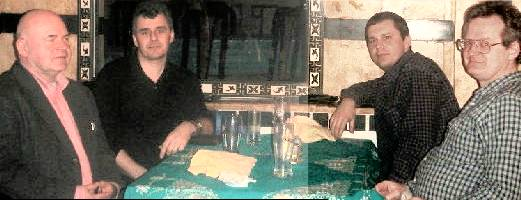42.Conditional moments of q-Meixner processes (with J. Wesolowski) [Corrected Version](Theorem 4.2) Probab. Theory Rel. Fields 131 (2005), 415-441.
43. Spectral measure of large random Hankel, Markov and Toeplitz matrices (with A. Dembo and T. Jiang) Ann. Probab. 34 (2006), 1-38. Expanded version from ArXiv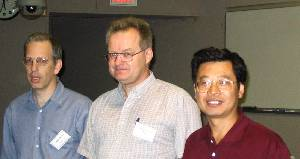44. On a class of free Levy laws related to a regression problem, (with M. Bozejko) Journal of Functional Analysis 236 (2006), 59-77.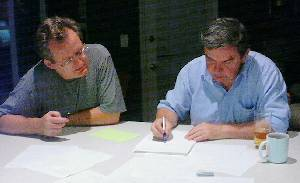45. The classical bi-Poisson process: an invertible quadratic harness (with J. Wesolowski) Statistics & Probability Letters 76 (2006), 1664-1674
46. Bi-Poisson Process (with J. Wesolowski), Infinite Dimensional Analysis, Quantum Probability and Related Topics, Vol. 10, No. 2 (2007) 277-291.
47.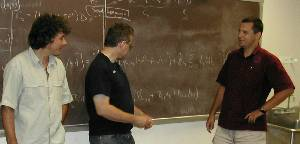Quadratic harnesses, q-commutations, and orthogonal martingale polynomials (with W. Matysiak and J. Wesolowski) Trans. Amer. Math. Soc. 359 (2007), no. 11, 5449--5483.
48. Computing moments of free additive convolution of measures Applied Mathematics and Computation, 194 (2007), 561-567.
49. The bi-Poisson process: a quadratic harness (with W. Matysiak and J. Wesolowski) Ann. Probab., 36 (2008), 623-646.
50.On slow-fading MIMO systems with non-separable correlation (with R. Rashidi Far, T. Oraby, and R. Speicher) IEEE Transactions on Information Theory, Volume 54, Issue 2, Feb. 2008, pp 544-553.
51. Asymptotic results for empirical measure of weighted sums of independent random variables (with B. Bercu) Electronic Comm. Probab. 12 (2007) 184--199. Erratum: Proof of Theorem 1 is incorrect: Lemma 2 is misquoted! (Noticed by Mark Meckes in 2010. Thank you Mark.).
52. Asymptotic normality for traces of polynomials in independent complex Wishart matrices Probab. Theor. Rel. Fields, 140 (2008) 383-405
53. Compound real Wishart and q-Wishart matrices. Intern. Math. Research Notices 2008: rnn079-42.
54.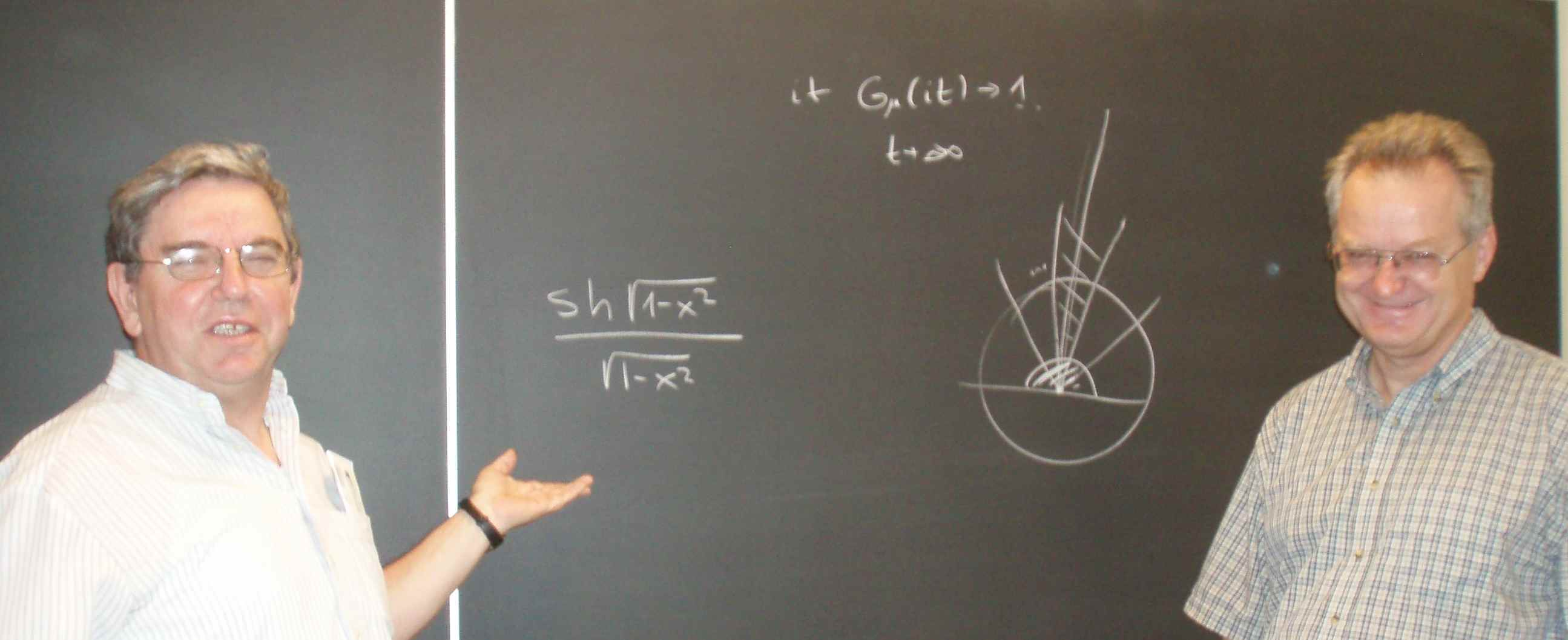A quadratic regression problem for two-state algebras with application to the Central Limit Theorem (with M. Bozejko) Infinite Dimensional Analysis, Quantum Probability and Related Topics, 12 (2009), 231-249.
55. Duality of real and quaternionic random matrices (with Virgil Pierce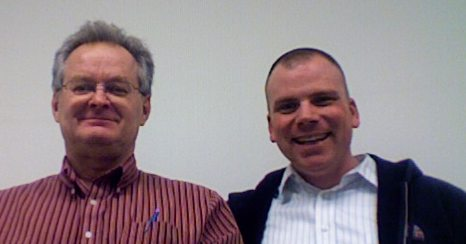) Electronic Journal Probab. Vol. 14 (2009), Paper no. 17, pages 452--476.
56. Free Exponential Families as Kernel Families Demonstr. Math., XLII (2009) 657-672 arxiv version
57.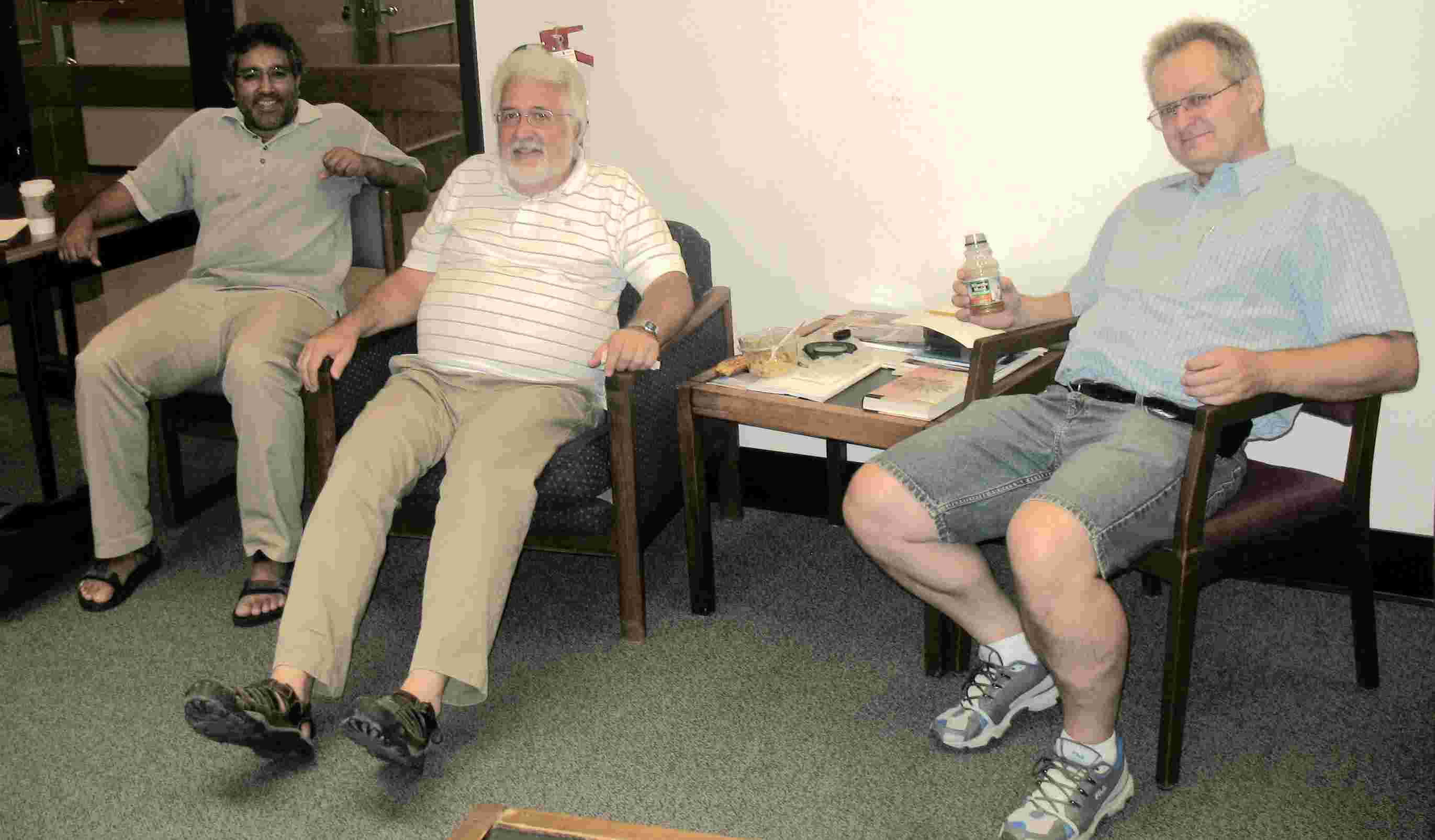Large deviations for the leaves in some random trees (with C. David Minda and Sunder Sethuraman) Advances in Applied Probab., 41 (2009), 845-873.
58. Askey-Wilson polynomials, quadratic harnesses and martingales (with Jacek Wesolowski) Ann. Probab., 38 (2010) pp. 1221--1262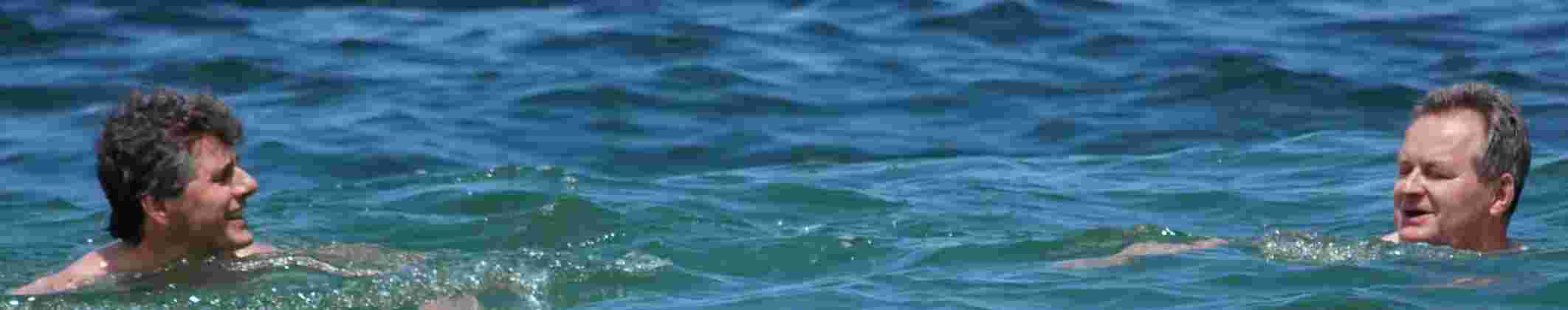59. A remark on the maximum eigenvalue for circulant matrices. (with Sunder Sethuraman) In: "High Dimensional Probabilities V: The Luminy Volume", IMS Collections Vol. 5 (2009) 179-184.60. Markov processes with free Meixner laws Stoch. Proc. Appl., 120 (2010) pp. 1393--1403.
61. Free Quadratic Harness (with Wojciech Matysiak, and Jacek Wesolowski), Stoch. Proc. Appl. 121 (2011) 657-671
62. One-sided Cauchy-Stieltjes Kernel Families (with Abdelhamid Hassairi) Journ. Theoret. Probab. 24 (2011) pp. 577--594.63. Bridges of quadratic harnesses (with Jacek Wesolowski) Electron. J. Probab. 17 (2012), no. 105, 1-25.64. Quadratic harnesses from generalized beta integrals in "Noncommutative Harmonic Analysis with Applications to Probability III", ed. M. Bozejko, A. Krystek, L. Wojakowski. Banach Center Publications Vol 96 (2012) pp. 67-79.
65. Stitching pairs of Levy processes into Harnesses, with Jacek Wesolowski. Stoch. Proc. Appl. Volume 122 (2012) pp. 2854-2869
66. On integrability of quadratic harnesses. Demonstratio Mathematica 45 (2012) 275--282.
67. Meixner matrix ensembles (with Gerard Letac) Journ. Theoret. Probab. 26 (2013) 107-152.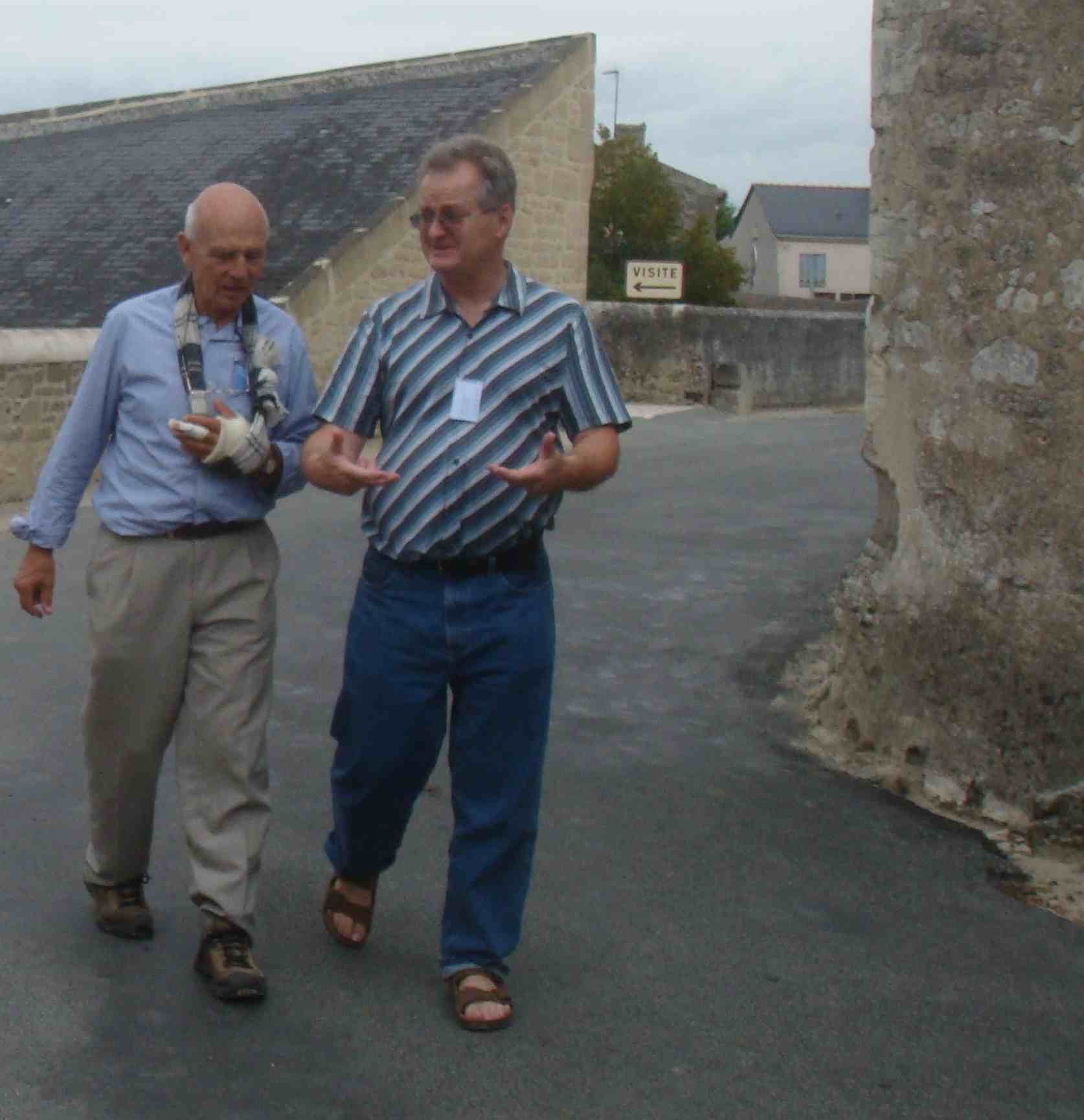68. Fock space realizations of some classical Markov processes, with Wiktor Ejsmont. Probability and Mathematical Statistics Vol. 33, (2013), pp. 301-313.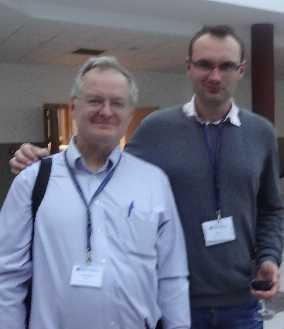69. Separation of the largest eigenvalues in eigenanalysis of genotype data from discrete subpopulations (with Kasia Bryc and Jack Silverstein) Theoretical Population Biology 89 (2013) 34-43. Corrected version (Oct 2017 - Corrected values M=120 N=2500 in numerical example in Section 3.1 and new proof of Lemma 2.)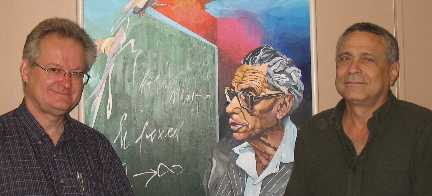70. On Cauchy-Stieltjes Kernel Families, with Raouf Fakhfakh and Abdelhamid Hassairi. Journ. Mutlivariate Anal. Vol. 124 (2014) pp. 296-312.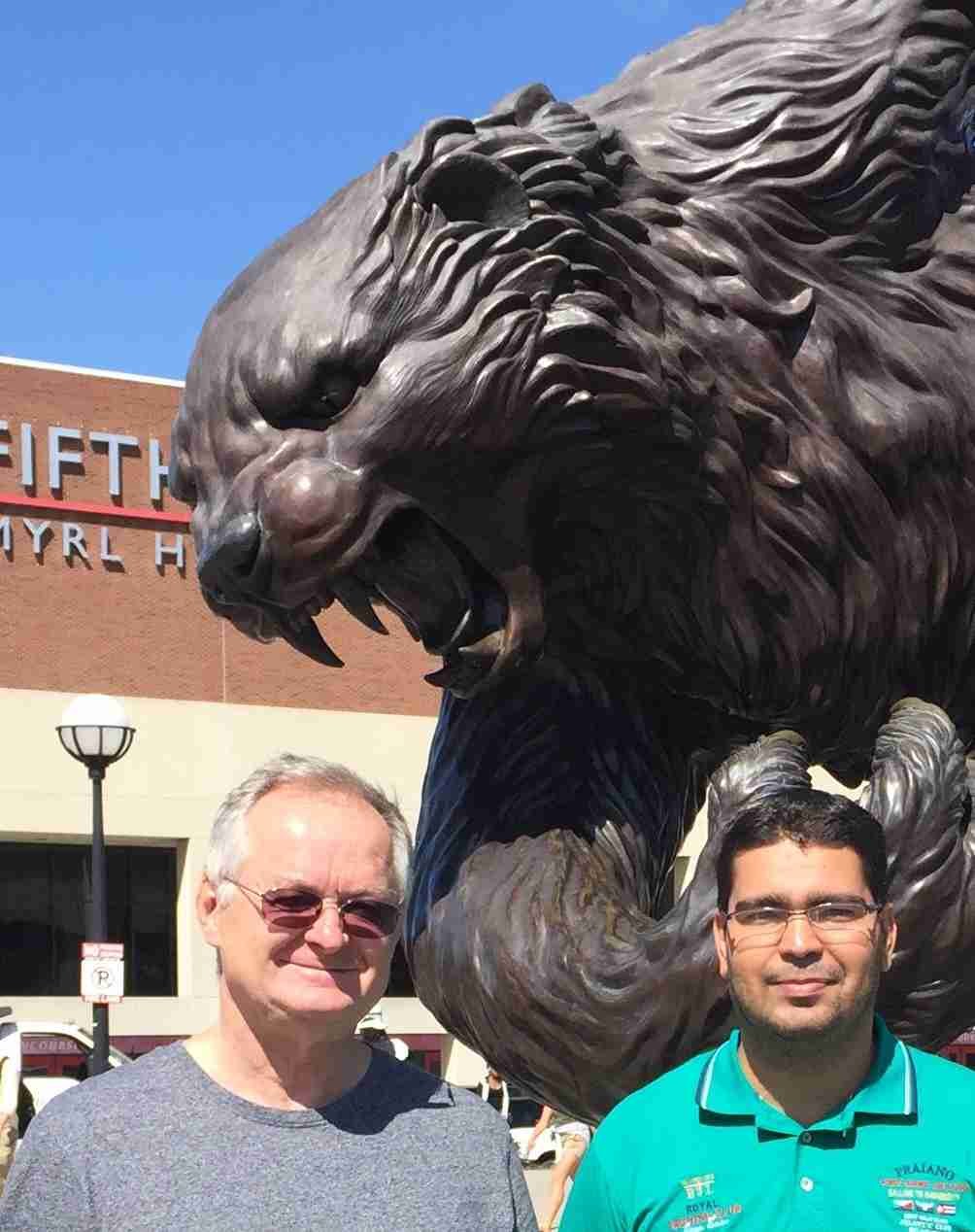71. Infinitesimal generators of q-Meixner Markov processes with Jacek Wesolowski, Stochastic Processes and their Applications 124/1 (2014), pp. 915-926
72. On integration with respect to the $q$-Brownian motion Stats Probab Letters 94(2014) 257-266
73. Wilson's 6-j laws and stitched Markov processes, with Wojciech Matysiak. Teorya Veroyatn. Primen. 60 (2015) 333-356. http://dx.doi.org/10.4213/tvp4622
74. Infinitesimal generators for a class of polynomial processes with Jacek Wesolowski Studia Mathematica 229 (2015), 73-93.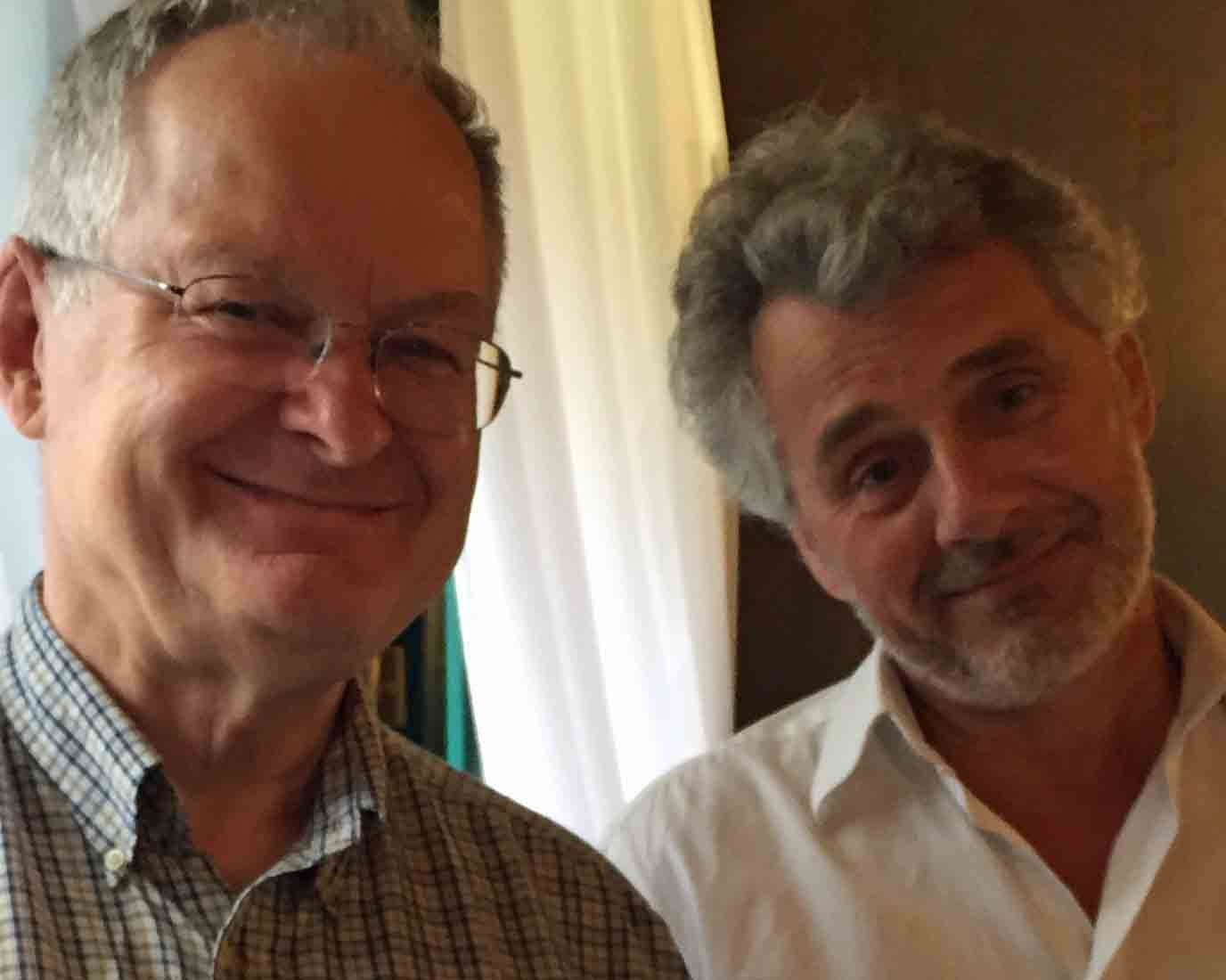75. The local structure of $q$-Gaussian processes, with Yizao Wang, Probab. Math. Stat. 36 (2016) pp. 335-352.76. Asymmetric Simple Exclusion Process with open boundaries and Quadratic Harnesses, with Jacek Wesolowski Journal of Statistical Physics 167 (2017), 383-415. DOI: 10.1007/s10955-017-1747-5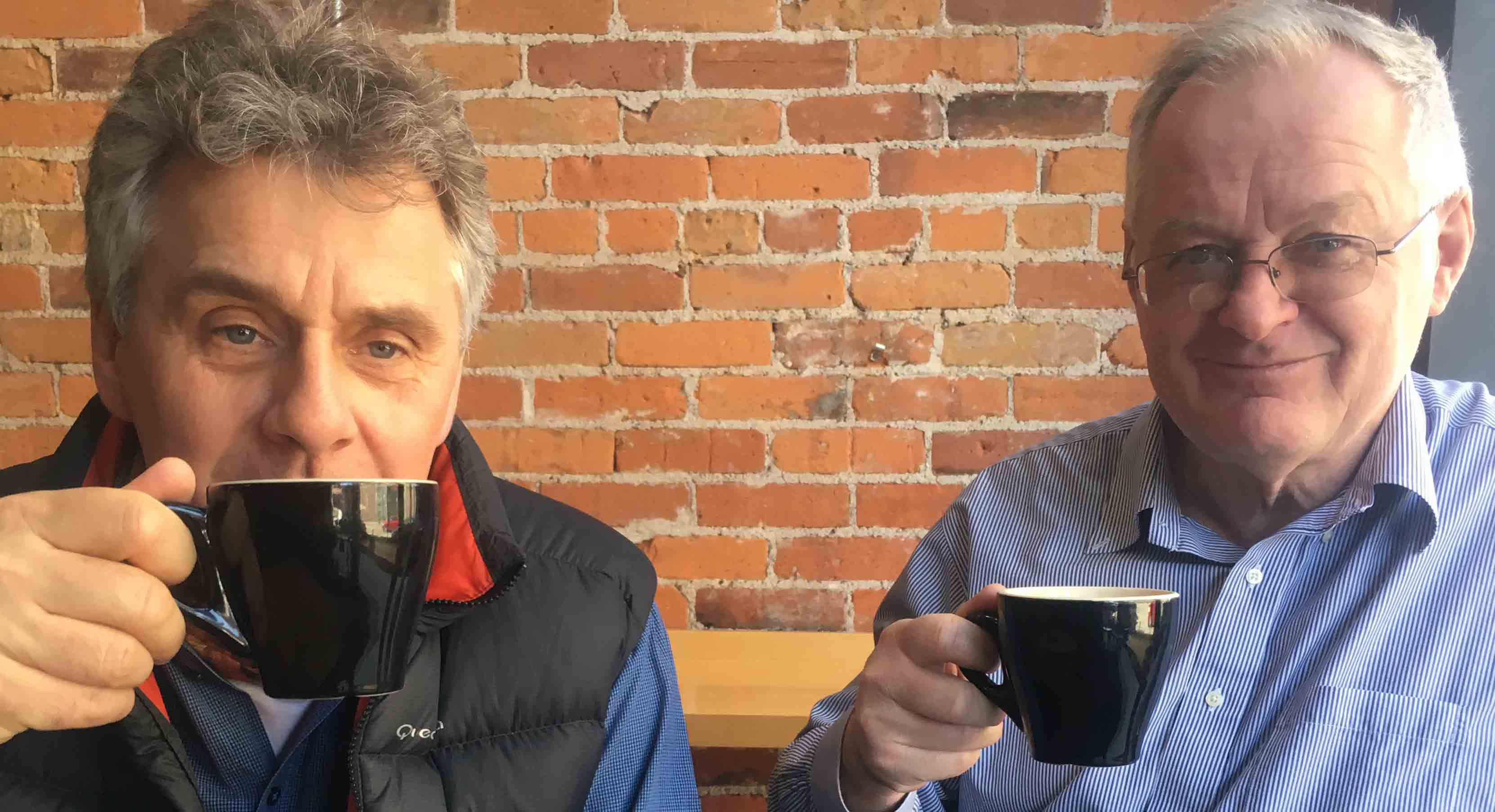77. Dual representations of Laplace transforms of Brownian excursion and generalized meanders, with Yizao Wang Statistics and Probability Letters 140 (2018) 77-83.
78. Limit fluctuations for density of asymmetric simple exclusion processes with open boundaries, with Yizao Wang. Annales de l'Institut Henri Poincare 55 (2019) pp. 2169–2194. https://doi.org/10.1214/18-AIHP945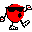Prize79. Cauchy-Stieltjes families with polynomial variance functions and generalized orthogonality, with Raouf Fakhfakh and Wojciech Mlotkowski. Probability and Mathematical Statistics 39 (2019) pp 237-258. PDF https://doi.org/10.19195/0208-4147.39.2.1
80. Fluctuations of random Motzkin paths, with Yizao Wang Advances in Applied Mathematics 106 (2019) 96-116, https://doi.org/10.1016/j.aam.2019.02.003
81. On matrix product ansatz for Asymmetric Simple Exclusion Process with open boundary in the singular case with Marcin Swieca, Journal of Statistical Physics, 177 (2019), pp. 252-284 https://doi.org/10.1007/s10955-019-02367-4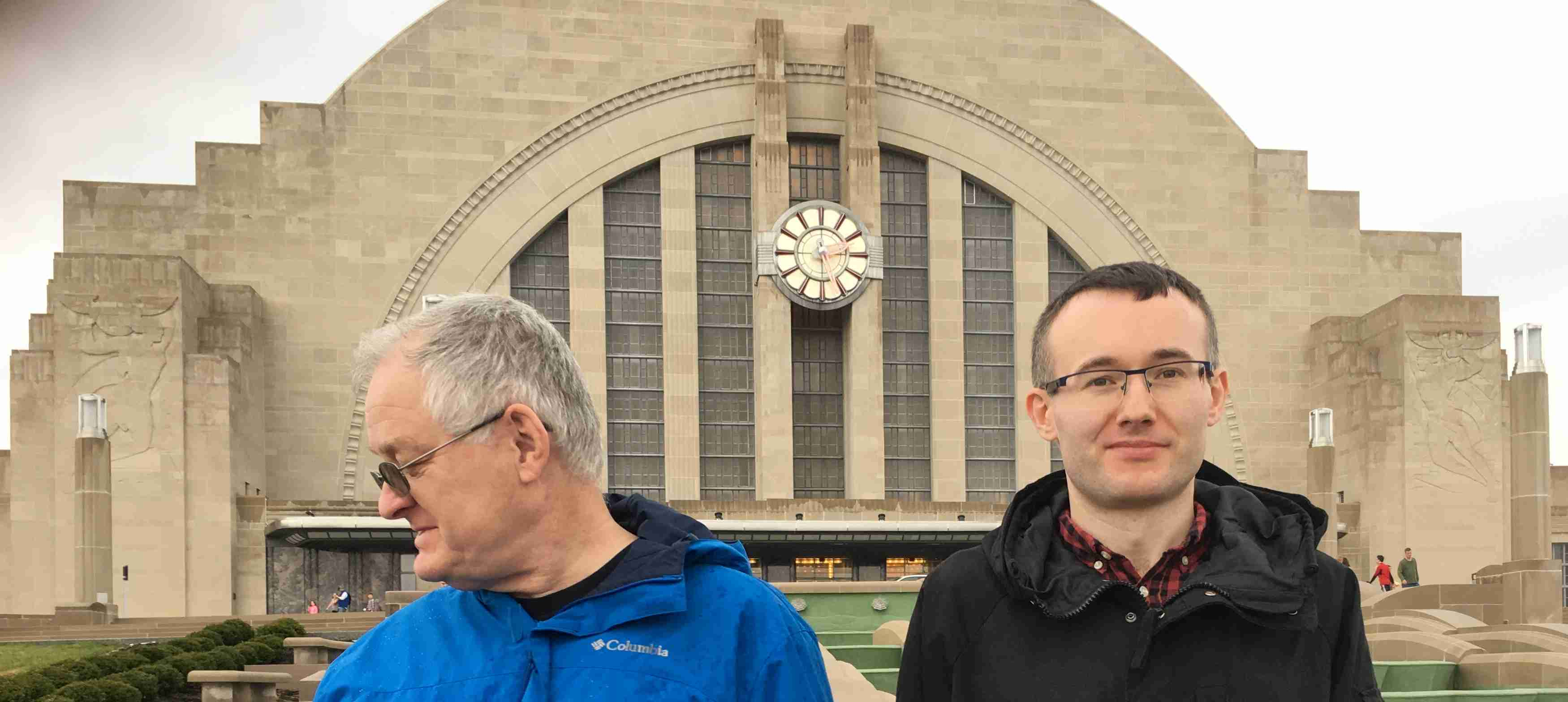82. Singular values of large non-central random matrices, with Jack Silverstein, Random Matrices: Theory and Applications, Vol. 9, (2020) 2050012 (32 pages) https://doi.org/10.1142/S201032632050012483. Flows in near algebras with probabilistic applications, with Jacek Wesolowski and Agnieszka Zieba#### Preprint form only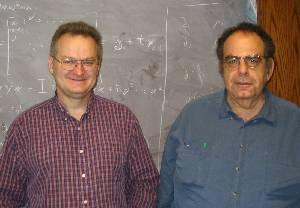1. Approximation Operators, Exponential, and q-Exponential Families (with M. Ismail) [Dec 2005]
2. Projection formulas for orthogonal polynomials (with W. Matysiak, R. Szwarc, and J. Wesolowski3. Spectra of large block matrices (with T. Oraby, R. Rashidi Far, and R. Speicher) 

#### Other Papers

1. O intensywnosci uszkodzen elementow dzialajacych zaleznie (with J. Rudnicki) in Polish, Zag. Ekspl. Maszyn 1979
2. Decoding a scrambled text : a hands-on project to illustrate Sampling and Variability Journal Statist. Education, Volume 7, Number 2 (July 1999) .
3. A uniform approximation to the right normal integral, Applied Mathematics and Computation, 127 (2002), pp. 365-374. with online-only Addendum